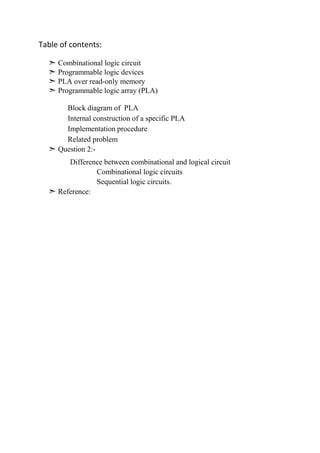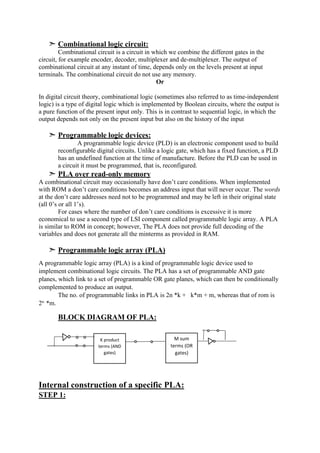Diese Präsentation wurde erfolgreich gemeldet.

# Programmable Logic Array(PLA), digital circuits

Anzeige
Anzeige
Anzeige
Anzeige
Anzeige
Anzeige
Anzeige
Anzeige
Anzeige
Anzeige
AnzeigeAnzeige
×

1 von 9 Anzeige

# Programmable Logic Array(PLA), digital circuits

this assignmwnt states difference between combinational and sequential circuits, explain PLA and compare it with ROM

this assignmwnt states difference between combinational and sequential circuits, explain PLA and compare it with ROM

Anzeige
Anzeige

### Programmable Logic Array(PLA), digital circuits

1. 1. Submitted by: Warda Aziz (101631058) Submitted to: Miss Amna Dilawar Date of submission Subject title: Digital logic and comupter designing Govt. PostGraduate ColleGe for Women samnabd, lahore
2. 2. Table of contents: ➣ Combinational logic circuit ➣ Programmable logic devices ➣ PLA over read-only memory ➣ Programmable logic array (PLA) Block diagram of PLA Internal construction of a specific PLA Implementation procedure Related problem ➣ Question 2:- Difference between combinational and logical circuit Combinational logic circuits Sequential logic circuits. ➣ Reference:
3. 3. ➣ Combinational logic circuit: Combinational circuit is a circuit in which we combine the different gates in the circuit, for example encoder, decoder, multiplexer and de-multiplexer. The output of combinational circuit at any instant of time, depends only on the levels present at input terminals. The combinational circuit do not use any memory. Or In digital circuit theory, combinational logic (sometimes also referred to as time-independent logic) is a type of digital logic which is implemented by Boolean circuits, where the output is a pure function of the present input only. This is in contrast to sequential logic, in which the output depends not only on the present input but also on the history of the input ➣ Programmable logic devices: A programmable logic device (PLD) is an electronic component used to build reconfigurable digital circuits. Unlike a logic gate, which has a fixed function, a PLD has an undefined function at the time of manufacture. Before the PLD can be used in a circuit it must be programmed, that is, reconfigured. ➣ PLA over read-only memory A combinational circuit may occasionally have don’t care conditions. When implemented with ROM a don’t care conditions becomes an address input that will never occur. The words at the don’t care addresses need not to be programmed and may be left in their original state (all 0’s or all 1’s). For cases where the number of don’t care conditions is excessive it is more economical to use a second type of LSI component called programmable logic array. A PLA is similar to ROM in concept; however, The PLA does not provide full decoding of the variables and does not generate all the minterms as provided in RAM. ➣ Programmable logic array (PLA) A programmable logic array (PLA) is a kind of programmable logic device used to implement combinational logic circuits. The PLA has a set of programmable AND gate planes, which link to a set of programmable OR gate planes, which can then be conditionally complemented to produce an output. The no. of programmable links in PLA is 2n *k + k*m + m, whereas that of rom is 2n *m. BLOCK DIAGRAM OF PLA: Internal construction of a specific PLA: STEP 1: K product terms (AND gates) M sum terms (OR gates)
4. 4. STEP 2: The next part is to draw a vertical wire with an AND gate STEP 3: The first AND gate (on the left) is the min term: x2’.x1’.x0 The second AND gate (from the left) is the minterm: x2’.x1.x0 The third AND gate (from the left) is the minterm: x2’.x1’.x0’ I’ve added a fourth AND gate which is the minterm: x2.x1.x0. STEP 4: We then add OR gates using horizontal wires, to connect the minterms together. Implementation procedure 1. Preparation in SOP (sum of products) form. 2. Obtain the minimum SOP form to reduce the number of product terms to a minimum. 3. Decide the input connection of the AND matrix for generating the required product term.
5. 5. 4. Then decide the input connections of OR matrix to generate the sum terms. 5. Decide the connections of invert matrix. 6. Program the PLA. Let’s try to implement these function f1 and f2 are given as Inputs x1, x2, x3 and their respective complemented signals are given to programmable AND plane, there we will get AND plane outputs as P1, P2, P3called minterms. Then these signals are given to programmable OR plane to produce required output function f1 and f2 (sum of products). The below figure describes the gate level implementation of the PLA for given functionality.
6. 6. Related problem: Derive the PLA program table for a combinational circuit that squares s 3-bit number. Solution: PLA program table: A B C F1 F2 F3 F4 0 0 0 0 0 0 0 0 0 1 0 0 0 0 0 1 0 0 0 0 1 0 1 1 0 0 1 0 1 0 0 0 1 0 0 1 0 1 0 1 1 0 1 1 0 1 0 0 1 1 1 1 1 1 0 0 K-map for F1: B’C’ B’C BC BC’ A 1 1 A’ K-map for F1’: B’C’ B’C BC BC’ A 0 0 A’ 0 0 0 0 F1= AB F1’=A’+B’ K-map for F2: B’C’ B’C BC BC’ A 1 1 1 A’ K-map for F2’: B’C’ B’C BC BC’ A 0 A’ 0 0 0 0 F2= AB’+AC F2’=A’+BC’ K-map for F3: B’C’ B’C BC BC’ A 1 A’ 1
7. 7. K-map for F3’: B’C’ B’C BC BC’ A 0 0 0 A’ 0 0 0 F3= AB’C+A’BC F3’=A’B’+AB+C’ K-map for F4: B’C’ B’C BC BC’ A 1 A’ 1 K-map for F4: B’C’ B’C BC BC’ A 0 0 0 1 A’ 0 0 0 1 F4= BC’ F4’=B’+C PROGRAM TABLE: A B C F1 F2 F3 F4 AB 1 1 - 1 - - - A’ 0 - - - 1 - - BC’ - 1 0 - 1 - 1 A’B’ 0 0 - - - 1 - C’ - - 0 - - 1 - T C C T Question 2:- Difference between combinational and logical circuit Combinational logic circuits. The simple time independent logic circuits that are implemented using Boolean circuits whose output logic value depends only on the input logic values can be called as combinational logic circuits.
8. 8. Sequential logic circuits. The simple logic circuits whose output logic value depends on the input logic values and also on the stored information is called as sequential logic circuits. Major differences: Combinational logic circuits Sequential logic circuits These combinational digital logic circuits don’t have the capability to store a state inside them. These sequential digital logic circuits are capable to retain the earlier state of the system based on the current inputs and earlier state. the combinational logic circuits do not contain any memory elements The sequential logic circuits contain memory elements. The combinational digital logic circuits are fundamentally implemented using different types of devices such as multiplexers, de- multiplexers, encoders, decoders, half adder, and full adders. The latch is considered as the simplest element used to retain the earlier memory or state in the sequential digital logic. As there are no clocks used in these digital logic circuits, they do not need any triggering The maximum number of sequential logic circuits uses a clock for triggering the flip flops operation The combinational digital circuits don’t require any feedbacks The sequential digital logic circuits utilize the feedbacks from outputs to inputs.
9. 9. Reference: www.elprocus.com/sequential-circuit-design-using-pla/ http://www.edgefx.in/wp-content/uploads/2015/02/Combinational-Logic-Circuit.jpg Digital logic & computer design by Morris Mano